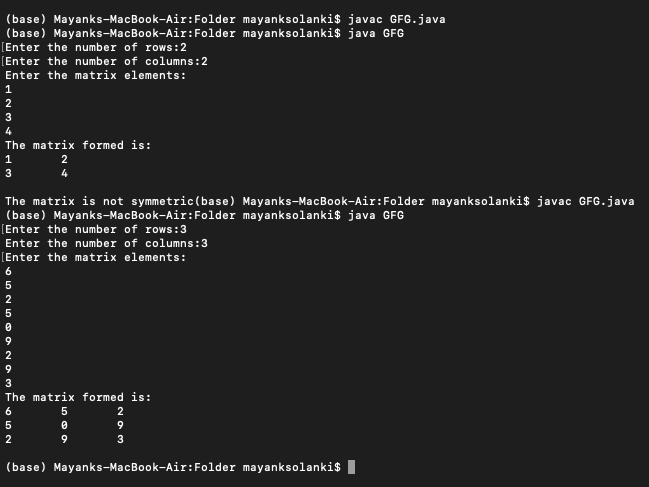# Java Program to Check Whether a Matrix is Symmetric or Not

• Last Updated : 03 Nov, 2021

A symmetric matrix is a square matrix in which the transpose of the square matrix is the same as the original square matrix. A square matrix is a matrix in which the number of columns is the same as the number of rows. If the matrix is a symmetric matrix then it is also a square matrix but vice versa isn’t true. Matrix is simply considered as 2D arrays for action to be performed. Here, we are going to see the approach to check if the entered square matrix is symmetric or not.

Illustration:

```Input : 6 5 2
5 0 9
2 9 3
Output: The matrix is symmetric

Input : 6 1 2
5 0 9
2 9 3
Output: The matrix is not symmetric```

Approach:

• Take the matrix as an input from the user
• Find transpose of the matrix
• Compare two matrices
• If the two matrices is the same then it is symmetric otherwise it’s not.

Implementation:

Example

## Java

 `// Java Program to check whether matrix is``// symmetric or not` `// Importing all classes of``// java.util package``import` `java.util.*;` `// Class``public` `class` `GFG {``    ``// Matrix 1` `    ``// Method to check whether the matrix is``    ``// symmetric or asymmetric``    ``static` `void` `checkSymmetric(``int` `mat[][], ``int` `row,``                               ``int` `col)``    ``{``        ``int` `i, j, flag = ``1``;` `        ``// Display message``        ``System.out.println(``"The matrix formed is:"``);` `        ``// Nested for loop for matrix iteration` `        ``// Outer loop for rows``        ``for` `(i = ``0``; i < row; i++) {``            ``// Inner loop for columns``            ``for` `(j = ``0``; j < col; j++) {``                ``// Print matrix``                ``System.out.print(mat[i][j] + ``"\t"``);``            ``}` `            ``System.out.println(``""``);``        ``}` `        ``// Matrix 2` `        ``// Finding transpose of the matrix``        ``int``[][] transpose = ``new` `int``[row][col];` `        ``// Again, nested for loop for matrix iteration` `        ``// Outer loop for rows``        ``for` `(i = ``0``; i < row; i++) {` `            ``// Inner loop for columns``            ``for` `(j = ``0``; j < col; j++) {` `                ``// Print matrix elements``                ``transpose[j][i] = mat[i][j];``            ``}``        ``}` `        ``// Condition check over Matrix 1 with Matrix 2` `        ``if` `(row == col) {` `            ``// Outer loop for rows``            ``for` `(i = ``0``; i < row; i++) {` `                ``// Inner loop for columns``                ``for` `(j = ``0``; j < col; j++) {` `                    ``// Comparing two matrices``                    ``if` `(mat[i][j] != transpose[i][j]) {``                        ``flag = ``0``;``                        ``break``;``                    ``}``                ``}` `                ``// Setting a flag value for symmetric matrix``                ``if` `(flag == ``0``) {` `                    ``// Display message``                    ``System.out.print(``                        ``"\nThe matrix is not symmetric"``);``                    ``break``;``                ``}``            ``}` `            ``// Setting a flag value different from above``            ``// for symmetric matrix``            ``if` `(flag == ``1``) {` `                ``// Display message``                ``System.out.print(``                    ``"\nThe matrix is symmetric"``);``            ``}``        ``}` `        ``// If it isn't a square matrix``        ``// then it can't be a symmetric matrix``        ``else` `{` `            ``// Display message``            ``System.out.print(``                ``"\nThe matrix is not symmetric"``);``        ``}``    ``}` `    ``// Main driver method``    ``public` `static` `void` `main(String args[])``    ``{``        ``// Taking input from the user``        ``Scanner sc = ``new` `Scanner(System.in);` `        ``// Declaring variables and setting flag to 1``        ``int` `i, j, row, col, flag = ``1``;` `        ``// Taking input from the user``        ``System.out.print(``"Enter the number of rows:"``);``        ``row = sc.nextInt();` `        ``// Display message``        ``System.out.print(``"Enter the number of columns:"``);` `        ``// Reading matrix elements individually using``        ``// nextInt() method``        ``col = sc.nextInt();` `        ``// Declaring a 2D array(matrix)``        ``int``[][] mat = ``new` `int``[row][col];` `        ``// Display message``        ``System.out.println(``"Enter the matrix elements:"``);` `        ``// Nested for loop for traversing matrix` `        ``// Outer loop for rows``        ``for` `(i = ``0``; i < row; i++) {` `            ``// Inner loop for columns``            ``for` `(j = ``0``; j < col; j++) {` `                ``// Print matrix element``                ``mat[i][j] = sc.nextInt();``            ``}``        ``}` `        ``// calling function made above to check``        ``// whether matrix is symmetric or not``        ``checkSymmetric(mat, row, col);``    ``}``}`

Output: Below both symmmericity and asymetricity is checked as follows:My Personal Notes arrow_drop_up